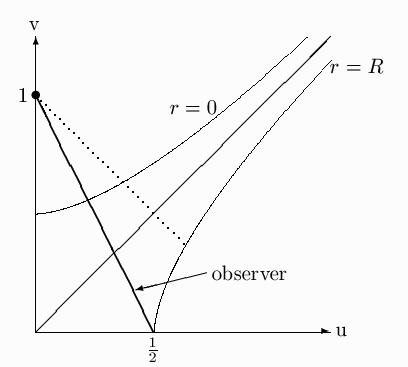Solutions set 8 12-5 Use conservation of e (l = 0). The observer's initial 4-velocity is u = [(1-2M/R)-½, 0, 0, 0 ] = [hÖ5, 0, 0, 0] (from u·u = -1), therefore e = (1-2M/R)ut = 2/Ö5. At other radii, using u·u = -1,
 ćč dr dt öř 2 = e2 - ćč 1 - 2M r öř = 2M r - 1 5
hence
 t = óő 10M 0 ćč 2M r - 1 5 öř -1/2 .
which works out to be (5Ö5)pM.

12-15 At ejection, energy-momentum is conserved, but not necessarily rest mass. Let p be the original 4-momentum, pL that of the eject, and pe the escaping 4-momentum, then p = pL + pe. The escaping mass receives the greatest energy if the ejected-into-black-hole matter is lightlike, so

pL2 = 0 = (p - pe)2 = p2 - 2p·pe + pe2.
But p2 = -m2,  pe2 = -me2; also p ·pe = mut gtt meuet. Now note gttuet = uet = (/t)·ue = (Killing vector)·ue = -e is conserved. If the escaping spaceship arrives at infinity with zero velocity, this conserved quantity has the value -1. Also, since the original spaceship was stationary, ut = (1-2M/R)-½. Putting this all together we get a quadratic equation for f = me/m:
1 - 2 f (1-2M/R)-½ + f2 = 0
with the solution
 f = 1 ±(2M/R)½ (1 - 2M/R)½
Only the - sign makes sense, since we know f < 1. (The + sign gives 1/f.) So f vanishes when R = 2M.

12-22
a)b) The straight line has a slope of 2 which means the observer is within the 45° lines that are the light cones.
c)The latest time is the value of t at which the 45° dotted line from u = 0, v = 1 intersects the curve r=R. The equation for the line is v = 1 - u. Substitute from (12.13a) with r = R, use the given expression for R, and solve for t to find t = 4M log(2).

24-6. Incompressible matter would imply an infinite speed of sound, inconsistent with SR.
Integrate (23.7) with constant r:

M(r) = 4/3 pr3 r
and insert in (23.7):
 - dp (r+ p)(r/3 + p) = 4pr3 dr r2(1- 8pr2/3) .
Integrate this with the boundary condition p(R) = 0 to find
 p(r) = r éë (1-8pr2/3)½ - (1 - 8prR2/3)½ 3(1-8pr2/3)½ - (1 - 8prR2/3)½ ůű .
There is a maximum mass M(r=R) because the pressure at the center becomes infinite when M/R = 4/9     (Mmax = 4/9(3pr)-½).

24-7. a) We need l(r) and n(r). From (24.12) with m(r) = 4/3 pr3 r, as in the previous problem, we get l(r). From (34.13c) we get
 1 2 dn dr = 8prr-½ 3 éë 3 ćč 1 - 8prR2 3 öř 1/2 - ćč 1 - 8prr2 3 öř 1/2 ůű -1
and this can be integrated, with the constant adjusted so as to match Schwarzschild at r = R, where
 n(R) = log ćč 1 - 2M R öř = log ćč 1 - 8prR2 3 öř .
The result is
 ds2 = - éë 3 2 ćč 1 - 8prR2 3 öř 1/2 - 1 2 ćč 1 - 8prr2 3 öř 1/2 ůű 2 dt2 + ćč 1 - 8prr2 3 öř -1 dr2 + r2(dq2 + sin2qdf2) .
b) The space part of the metric is (1 - const×r2)-1 dr2 + r2 dW2.
c) The volume inside the star is
 V = 4p óő R 0 r2 dr (1 - 8prr2/3)½ ł 4/3 pR3